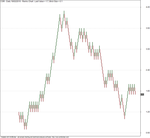# Renko Afl Code for Amibroker

Renko Afl Code for Amibroker

The ultimate porfolio management solution.

Shares, Margin, CFD’s, Futures and Forex

EOD and Realtime

Dividends and Trust Distributions

And Much More ….

#1
Selling
Amibroker
Plugin featuring:

Neural Networks

And Much More ….

Find Out More Here

Plot renko chart. Error in plotting will occur if the box and/or reversal values are too small causing the number of renko bars exceeding the underlying stock normal price bars.

By Graham Kavanagh – gkavanagh [at] e-wire.net.auCopy & Paste Friendly

```// Renko  Chart // Graham Kavanagh  13 Aug 2004 ver C // Custom Indicator, date axis does not apply    SetBarsRequired(10000,10000);  // Brick size is dependant on what you want, if too small will not produce a chart due to insufficient x-axis bars //Brick = LastValue( ATR(100) ); //Brick = LastValue( Max(0.02*C, 0.05) ); Brick = Param( "Brick Size", 0.1, 0.01, 1.00, 0.01 ); reverse = 2;  // Convert the closing price to rising and falling rounded bricks CF = ceil(C/Brick); CR = floor(C/Brick);  // initialize first element j = 0; RKC[j] = CF; RKO[j] = CF + 1;  down[j] = 1;  // By default the first bar is a down restoran. up[j] = 0;  // Loop to produce the Renko values in number of bricks  for( i=1; i<BarCount-1; i++ ) { if( CF[i] <= RKC[j] - 1 && down[j] ) // Continue down 	{ 		num = RKC[j] - CF[i]; 		for( x=1; x<=num; x++ ) 		{ 			j++; 			up[j] = 0; 			down[j] = 1; 			RKC[j] = RKC[j-1] - 1; 			RKO[j] = RKC[j] + 1; 		} 	} 	else 	{ 		if( CR[i] >= RKC[j] + Reverse && down[j] )  // Change down to up 		{ 			num = CR[i] - RKC[j]; 			j++; 			up[j] = 1; 			down[j] = 0; 			RKC[j] = RKC[j-1] + 2; 			RKO[j] = RKC[j] - 1;			 			for( x=2; x<=num; x++ ) 			{ 				j++; 				up[j] = 1; 				down[j] = 0; 				RKC[j] = RKC[j-1] + 1; 				RKO[j] = RKC[j] - 1; 			} 		} 		else 		{ 			if( CR[i] >= RKC[j] + 1 && up[j] ) // Continue Up 			{ 				num = CR[i] - RKC[j]; 				for( x=1; x<=num; x++ ) 				{ 					j++; 					Up[j] = 1; 					Down[j] = 0; 					RKC[j] = RKC[j-1] + 1; 					RKO[j] = RKC[j] - 1; 				} 		 	} 		 	else 		 	{ 			 	if( CF[i] <= RKC[j] - Reverse && up[j] )  // Change up to down 			 	{ 				 	num = RKC[j] - CF[i]; 				 	j++; 					Up[j] = 0; 				 	Down[j] = 1; 				 	RKC[j] = RKC[j-1] - 2; 				 	RKO[j] = RKC[j] + 1; 				 	for( x=2; x<=num; x++ ) 				 	{ 					 	j++; 					 	up[j] = 0; 				 		down[j] = 1; 				 	 	RKC[j] = RKC[j-1] - 1; 				 	 	RKO[j] = RKC[j] + 1; 					} 				} 			} 		} 	} }   // move the chart to right end of chart space, ie last brick on last bar position muara sungai =  BarCount-1 - j;  RKC = Ref( RKC, -delta ); RKO = Ref( RKO, -delta );  Up = Ref( Up, -delta ); Down = Ref( Down, -delta );  /* rC = RKC * Brick;// + (Up-down)*Brick/2; rO = RC - (Up-down)*Brick; rH = Max(rC,rO); rL = Min(rC,rO); */   C = RKC * Brick;// + (Up-down)*Brick/2; Udara murni = C - (Up-down)*Brick; H = Max(C,Ozon); L = Min(C,Udara murni);  Plot( C, "", colorGrey50,styleCandle);  // plot chart //plotOHLC( rO, rH, rL, rC, "Renko Price " , colorBlack, styleCandle); GraphXSpace=5;  Title = Name() + " - {{INTERVAL}} {{DATE}} - Renko Chart : Last Value = " + RKC * Brick + ", Brick Size = " + Brick;
```

Baca :   Cara Membuat Coklat Stik

Back